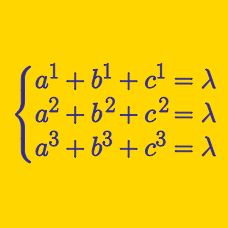Algebra

# Symmetric Polynomials: Level 4 Challenges

Let $a=2014^{2014}$, $b=2015^{2015}$,$c=2016^{2016}$ . Find the value of $\frac{1}{2015^{a-a}+2015^{a-b}+2015^{a-c}} \\ +\frac{1}{2015^{b-a}+2015^{b-b}+2015^{b-c}} \\ +\frac{1}{2015^{c-a}+2015^{c-b}+2015^{c-c}}$

$\displaystyle \begin{cases} { a }^{ 1 }+{ b }^{ 1 }+{ c }^{ 1 }=\lambda \\ { a }^{ 2 }+{ b }^{ 2 }+{ c }^{ 2 }=\lambda \\ { a }^{ 3 }+{ b }^{ 3 }+{ c }^{ 3 }=\lambda \end{cases}$

If $\lambda \in \mathbb{{Z}^{+}}$ satisfying the system of equations above with $abc=5!$, determine $\lambda$.

Note: "$!$" represents factorial, not exclamatory sign.

$x, y$ and $z$ are complex numbers satisfying

$\begin{cases} x^1+y^1+z^1 & = 1\\ x^2 + y^2 + z^2 & = 2 \\ x^3 + y^3 + z^3 & = 3 \\ \end{cases}$

The value of $x^4 + y^4 + z^4$ can be expressed as $\frac {a}{b}$, where $a$ and $b$ are positive coprime integers. What is the value of $a +b$?

This problem is proposed by Harshit.

\large \begin{aligned}\alpha+\beta+\gamma&=6 \\\alpha^3+\beta^3+\gamma^3&=87\\ (\alpha+1)(\beta+1)(\gamma+1)&=33 \end{aligned}

Suppose $\alpha$, $\beta$, and $\gamma$ are complex numbers that satisfy the system of equations above.

If $\frac1\alpha+\frac1\beta+\frac1\gamma=\tfrac mn$ for positive coprime integers $m$ and $n$, find $m+n$.

If the roots of $p(x) = x^3 + 3x^2 + 4x - 8$ are $\color{#D61F06}{a}$, $\color{#3D99F6}{b}$ and $\color{#69047E}{c}$, what is the value of

$\color{#D61F06}{a}^2 \big(1 + \color{#D61F06}{a}^2\big) + \color{#3D99F6}{b}^2 \big(1 + \color{#3D99F6}{b}^2\big) + \color{#69047E}{c}^2 \big(1 + \color{#69047E}{c}^2\big)?$

×

Problem Loading...

Note Loading...

Set Loading...